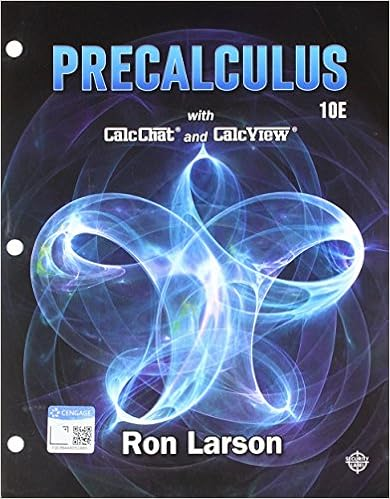# B4.08_Equation of a Parabola Based on Its Focus and Directrix_CA.doc

• Homework Help
• 5
• 71% (48) 34 out of 48 people found this document helpful

This preview shows page 1 - 2 out of 5 pages.

##### We have textbook solutions for you!
The document you are viewing contains questions related to this textbook.The document you are viewing contains questions related to this textbook.
Chapter 10 / Exercise 7
Precalculus
Hostetler/LarsonExpert Verified
© 2014 EDMENTUM, INC.Course ActivityEquation of a Parabola Based on Its Focus and DirectrixThis Course Activity will help you meet these educational goals:Content Knowledge—You will derive the equation of a parabola given a focus and directrix.Mathematical Practices—You will make sense of problems and solve them._________________________________________________________________________Directions and AnalysisWrite a response for each of the following activities. Check the Evaluation section at the end of this document to make sure you have met the expected criteria for the assignment. When you have finished, submit your work to your teacher.1.Deriving the Equation of a Parabola Given a Focus and Directrixa.The vertex form of the equation of a vertical parabola is given by 214yxhkpwhere (h, k) is the vertex of the parabola and the absolute value of pis the distance from the vertex to the focus, which is also the distance from the vertex to the directrix. You will use the GeoGebra geometry tool to create a vertical parabola and write the vertex form of its equation. Open GeoGebra, and complete each step below. If you need help, follow these instructionsfor using GeoGebra.i.Mark the focus of the parabola you are going to create at F(6, 4). Draw a horizontalline that is 6 units below the focus. This line will be the directrix of your parabola. What is the equation of the line?, Type your response here:
##### We have textbook solutions for you!
The document you are viewing contains questions related to this textbook.The document you are viewing contains questions related to this textbook.
Chapter 10 / Exercise 7
Precalculus
Hostetler/LarsonExpert Verified
•••